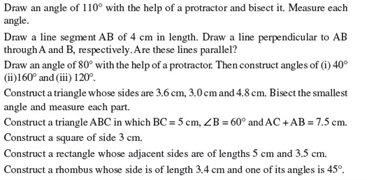# Revision Notes For CBSE Class 9 Math Chapter -11 Constructions

In geometry the term construction is mainly used for drawing different geometrical shapes like circle, triangle, square, rectangle, rhombus, parallelogram, etcaccurately using geometrical equipments including a ruler, pencil, compass and protractor. Each geometric shapes have their own construction steps and properties.

Here are the simple construction steps for drawing parallelogram ABCD.

Given: AB = 8 cm, BC = 9 cm and AC = 10 cm.

Step-1: With the help of a ruler and pencil, draw a straight line measuring AB =8 cm.

Step-2: Keeping C as midpoint, draw an arc BC = 9 cm.

Step-3: Now keeping A as center, draw an arc AC = 10 cm cutting the preceding arc at C.

Step-4: Connect AC and BC.

Step-5: Keeping C as middle and radius equal to 5 cm, draw an arc.

Step-6: With C as middle and radius equal to 10 cm, draw an arc cutting the previous arc at B.

Step-7: Now connect AD and CD.

Step-8:Here is the parallelogram ABCD.

Check here for the free CBSE Class 9 Math revision notes for Chapter 11- Constructions.